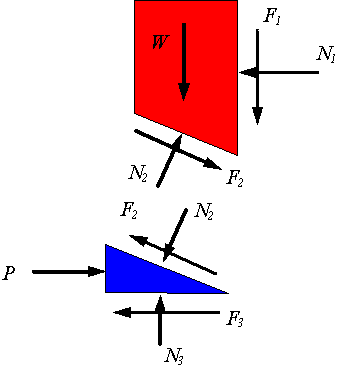# Wedges

## WEDGES

Wedges can quickly become very complicated to analyze. It is important to practice as many different problems as you can to ensure that you understand the principles behind these simple machines. Most importantly, you will always want to start with a free body diagram to illustrate the actual circumstance. I prefer to draw two, one for the actual circumstance and one for an "exploded" view (none of the components are touching). The figure below illustrates an exploded view. This exploded view allows for vectors to be drawn between the different components and also allows for analysis of one component at a time.Once you have drawn a neat and clear free body diagram it is now time to draw on your x/y axis. The purpose of the x/y axis is to allow for a mathematical analysis of the situation. Take your time to determine the best way to draw on your x/y axis. You will not calculate an incorrect answer if you choose the wrong x/y axis but you will do more work to get the answer. Wedges always have an incline between two or more surfaces so it will never be obvios which is the best way to draw your x/y axis.

The above figure is one of a series of figures that illustrates how to analyse wedges and screws. The following link is to the page that this illustration was taken from. It is advised to look over these images and, it you have time, read through it and try to follow the analysis.

## THE X/Y AXIS

Neither the above or below figures show an x/y axis; not very helpful. The figure below is a free body diagram of a small segment of a screw thread. The solid black arrow indicate where and how the actual forces are bieng applied. Can you see from the image what the author has chosen to be the x/y axis? Try it. Don't read on, try it. Don't read on, try it. Don't read on, try it. Okay so you can see that some of the vectors have been reduced to two orthogonal vectors. This is so all vectors are represented in only two planes; the x and y planes. The x plane is along the bottom incline of the component and the y plane, as always, is perpendicular to that. Now, using two equations that sum the forces in the x and y direction the problem can be solved.

If it can not be solved at this point it means that the equations have more unknowns variables than known variables. If this is the case, the equations need to be reduced until you have an amount of unknowns equal to or less than the number of equations (2). Your best bet is to see if you can describe on variable in terms of another (ie. friction = mu*N)